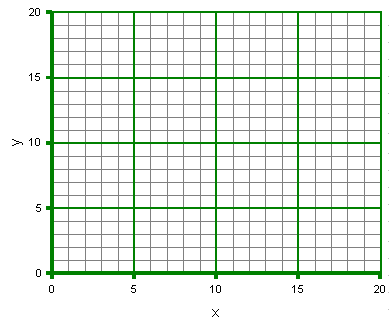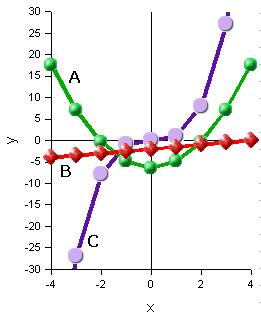### MS 095 PreAlgebra Quiz 03 • Name: __________

Plot the following data on the graph providedxy
14
47
109
1414
2017
1. _______________ Give an example of a monomial with a coefficient and a variable raised to the second power.
2. _______________ How many terms are in the expression 2.72x + 081?
3. _______________ What is the power of 5x3?
4. Simplify 5x + 7 - 5x2 + 7x - 13 - 2x2:

Matching

 ____ First degree equation y = mx + b____ Second degree equation y = ax2 + bx + c ____ Third degree equation y = ax3 + bx2 + cx + d